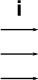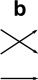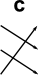# Maths - Alternating Groups

The alternating group is important from a mathematical point of view because, for A5 and above, it is a simple group which means it cannot be factored into smaller groups. It therefore plays an important pat in the categorization of groups.

The alternating group is a group containing only even permutations of the symmetric group. It turns out that half the permutations of the symmetric group are even and the other half are odd. That is if we permute a set with 'n' elements the symmetric group has !n permutations and the alternating group has !n/2 permutations.

### Even and Odd Permutations

Every permutation in a symmetric group (in other words, for every group) can be expressed as a product of 2-cycles. If the permutation has an even number of 2-cycles then it is an even permutation and if the permutation has an odd number of 2-cycles then it is an odd permutation.

In technical terms there is a short exact sequence:

An->Sn->C2

Sn is the semidirect product AnC2

### Example for A3

element
(permutation)
i a b c d e
S3This contains three 2-cycles as follows:

element
(permutation)
a b e
2-cyclesSo we can construct any permutation element from a sequence of 2-cycles like this:

permutation sequence of 2-cycles number of 2-cycles even or odd
i0 even
a1 odd
b1 odd
c2 even
d2 even
e1 odd

### Size of Alternating sets

number of elements of a set possible ways to order set |Sn| possible ways to order set |An|= |Sn| /2
1 1
2 2 1
3 6 3
4 24 12
n !n !n/2

### Signature or Cycle Shape

 symmetric group alternating group S1 () S2 () (1,2) S3 A3 () (2,3) (1,3) (1,2) (1,2,3) (1,3,2) S4 A4 () (3,4) (2,4) (1,4) (2,3) (1,3) (1,2) (1,2),(3,4) (2,3),(1,4) (1,3),(2,4) (1,3,4) (1,4,3) (2,3,4) (2,4,3) (3,1,2) (3,2,1) (4,1,2) (4,2,1) (1,2,3,4) (1,2,4,3) (1,3,2,4) (1,3,4,2) (1,4,2,3) (1,4,3,2) S5 A5 () (4,5) (3,5) (2,5) (1,5) (3,4) (2,4) (1,4) (2,3) (1,3) (1,2) (1,2),(3,4) (1,2),(4,5) (1,2),(3,5) (2,3),(1,4) (2,3),(1,5) (2,3),(5,4) (1,3),(2,4) (1,3),(2,5) (1,3),(5,4) (),() (2,3,4) (2,4,3) (1,3,4) (1,4,3) (4,1,2) (4,2,1) (3,1,2) (3,2,1) (1,2,3,4) (1,2,4,3) (1,3,2,4) (1,3,4,2) (1,4,2,3) (1,4,3,2) (1,2,3,4,5)
n An cycle notation Ancycle compressed Signature
2   <1> 12
3   <(1 2 3)> 13,3
4 <(), (123), (132), (124), (142), (134), (143), (234), (243), (12)(34), (13)(24), (14)(23)> <(1 2)(3 4),(1 2 3)> 14,3
5   <(3 4 5),(1 2 3)> 15,(22,1),(3,12),(5)
6   <(1 2)(3 4 5 6),(1 2 3)>
7   <(3 4 5 6 7),(1 2 3)>
8   <(1 2)(3 4 5 6 7 8),(1 2 3)>
9   <(3 4 5 6 7 8 9),(1 2 3)>
10   <(1 2)(3 4 5 6 7 8 9 10),(1 2 3)>

## Generating a Alternating Group using a Program

We can use a computer program to generate these groups, here I have used Axiom/FriCAS which is described here.

```a1 := alternatingGroup(1)

```
<1>
```

Type: PermutationGroup(Integer)
toTable()\$toFiniteGroup(a1,1)

```
 a a a a
```

Type: Table(2)
permutationRepresentation(a1,1)

```
[
 1
]
```

Type: List(Matrix(Integer))
a2 := alternatingGroup(2)

```
<1>
```

Type: PermutationGroup(Integer)
toTable()\$toFiniteGroup(a2,1)

```
 a a a a
```

Type: Table(2)
permutationRepresentation(a2,2)

```
[
 1 0 0 1
]
```

Type: List(Matrix(Integer))
a3 := alternatingGroup(3)

```
<(1 2 3)>
```

Type: PermutationGroup(Integer)
toTable()\$toFiniteGroup(a3,1)

```
 i a aa a aa i aa i a
```

Type: Table(3)
permutationRepresentation(a3,3)

```
[
 0 0 1 1 0 0 0 1 0
]
```

Type: List(Matrix(Integer))
a4 := alternatingGroup(4)

```
<(1 2)(3 4),(1 2 3)>
```

Type: PermutationGroup(Integer)
toTable()\$toFiniteGroup(a4,1)

```
 i a b ab ba bb aba abb bab bba babb bbab a i ab b aba abb ba bb bba bab bbab babb b ba bb bab bba i abb babb bbab a aba ab ab aba abb bba bab a bb bbab babb i ba b ba b bab bb abb babb bba i a bbab ab aba bb bba i bbab a b babb aba ab ba abb bab aba ab bba abb bb bbab bab a i babb b ba abb bab a babb i ab bbab ba b aba bb bba bab abb babb a bbab ba i ab aba b bba bb bba bb bbab i babb aba a b ba ab bab abb babb bbab ba aba b bab ab bba bb abb i a bbab babb aba ba ab bba b bab abb bb a i
```

Type: Table(12)
permutationRepresentation(a4,4)

```
[
 0 1 0 0 1 0 0 0 0 0 0 1 0 0 1 0
,
 0 0 1 0 1 0 0 0 0 1 0 0 0 0 0 1
]
```

Type: List(Matrix(Integer))
a5 := alternatingGroup(5)

```
<(3 4 5),(1 2 3)>
```

Type: PermutationGroup(Integer)
order(a5)

```
60
```

Type: PositiveInteger
permutationRepresentation(a5,5)

```
[
 1 0 0 0 0 0 1 0 0 0 0 0 0 0 1 0 0 1 0 0 0 0 0 1 0
,
 0 0 1 0 0 1 0 0 0 0 0 1 0 0 0 0 0 0 1 0 0 0 0 0 1
]
```

Type: List(Matrix(Integer))
a6 := alternatingGroup(6)

```
<(1 2)(3 4 5 6),(1 2 3)>
```

Type: PermutationGroup(Integer)
order(a6)

```
360
```

Type: PositiveInteger
permutationRepresentation(a6,6)

```
[
 0 1 0 0 0 0 1 0 0 0 0 0 0 0 0 0 0 1 0 0 1 0 0 0 0 0 0 1 0 0 0 0 0 0 1 0
,
 0 0 1 0 0 0 1 0 0 0 0 0 0 1 0 0 0 0 0 0 0 1 0 0 0 0 0 0 1 0 0 0 0 0 0 1
]
```
Type: List(Matrix(Integer))
(19) ->
```

where:

• The points of the permutation are numbered 1..n
• The elements of the group are named: "i" for the identity, single letters "a","b"... for the generators, and products of these.
• numbers in brackets are points of permutations represented in cyclic notation.
• The permutation is represented by a set of comma seperated permutations in angle brackets like this: <(1 2)(3 4),(1 2 3)>
• non-changing elements of the permutation are ommited so the above case is equivalent to: <(1 2)(3 4),(1 2 3)(4)>
• attempting to produce a alternating group with less than 3 elements does not produce a valid result.
• The Axiom/FriCAS program can't work in terms of the Cayley table, so I have added my own code to do this.Symmetry and the Monster - This is a popular science type book which traces the history leading up to the discovery of the largest symmetry groups.